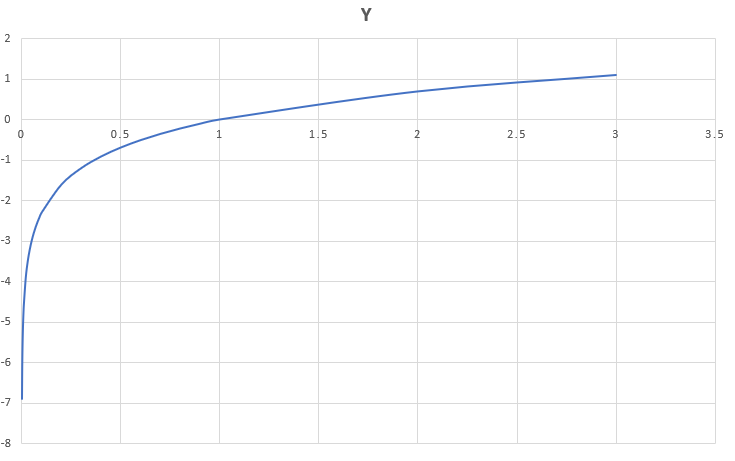# Performing Logarithmic Computations in R Programming – log(), log10(), log1p(), and log2() Functions

`log()` function in R Language returns the natural logarithm (base-e logarithm) of the argument passed in the parameter.

Syntax: log(x)

Parameter:
x: Specified value.

Returns: It returns the natural logarithm of the specified value, infinity for 0 and NaN for the negative value.

Example 1:

 `# R program to calculate log value  ` `print``(log(``1``))  ` `print``(log(``30``))  ` `print``(log(``0``))  ` `print``(log(``-``44``))  `

Output:

``` 0
 3.401197
 -Inf
 NaN
Warning message:
In log(-44) : NaNs produced
```#### log(x, base = y)

The `log(x,base=y)` is an inbuilt function in R which is used to compute the logarithm of the specified value to base y, infinity for 0, and NaN for the negative value.

Syntax: log(x, base = y)

Parameters:
x and base y.

Returns: It returns the logarithm of the specified value to base y, infinity for 0, and NaN for the negative value.

Example 2:

 `# R program to calculate log value  ` `print``(log(``10``,base``=``10``))  ` `print``(log(``16``,base``=``2``))  ` `print``(log(``0``,base``=``10``))  ` `print``(log(``-``44``,base``=``4``))  `

Output:

``` 1
 4
 -Inf
 NaN
Warning message:
In print(log(-44, base = 4)) : NaNs produced
```

#### log10() Function

The `log10()` is an inbuilt function in R which is used to compute the logarithm of the specified value to base 10, infinity for 0, and NaN for the negative value.

Syntax: log10(x)

Parameters:
x: Specified values.

Returns: It returns the logarithm of the specified value to base 10,infinity for 0 and NaN for negative value.

Example 2:

 `# R program to calculate log value  ` `print``(log10(``1``))  ` `print``(log10(``10``))  ` `print``(log10(``0``))  ` `print``(log10(``-``44``))  `

Output:

``` 0
 1
 -Inf
 NaN
Warning message:
In print(log10(-44)) : NaNs produced
```

#### log1p()

The `log1p()` is an inbuilt function in R which is used to calculate accurate natural logarithm of 1+x, where x is the specified value and throws infinity for 0 and NaN for negative value.

Syntax: log1p(x)

Parameters:
x: Specified values.

Returns: It returns the accurate natural logarithm of 1+x, where x is the specified value and throws infinity for 0 and NaN for negative value.

Example:

 `# R program to illustrate  ` `# the use of log1p() method ` ` `  `# Getting the accurate natural  ` `# logarithm of 1 + x, where x is  ` `# the specified value and throws  ` `# infinity for 0 and NaN for negative value. ` `print``(log1p(``1``)) ` `print``(log1p(``10``)) ` `print``(log1p(``0``)) ` `print``(log1p(``-``44``)) `

Output:

``` 0.6931472
 2.397895
 0
 NaN
Warning message:
In log1p(-44) : NaNs produced
```

#### log2()

The `log2()` is an inbuilt function in R which is used to calculate the logarithm of x to base 2, where x is the specified value or throws infinity for 0 and NaN for negative value.

Syntax: log2(x)

Parameters:
x: Specified values.

Returns: It returns the logarithm of x to base 2, where x is the specified value or throws infinity for 0 and NaN for negative value.

Example:

 `# R program to illustrate  ` `# the use of log2() method ` ` `  `# Getting the logarithm of x  ` `# to base 2, where x is the  ` `# specified value or throws  ` `# infinity for 0 and NaN for negative value. ` `print``(log2(``1``)) ` `print``(log2(``2``)) ` `print``(log2(``0``)) ` `print``(log2(``-``44``)) `

Output:

``` 0
 1
 -Inf
 NaN
Warning message:
In print(log2(-44)) : NaNs produced
```

My Personal Notes arrow_drop_upCheck out this Author's contributed articles.

If you like GeeksforGeeks and would like to contribute, you can also write an article using contribute.geeksforgeeks.org or mail your article to contribute@geeksforgeeks.org. See your article appearing on the GeeksforGeeks main page and help other Geeks.

Please Improve this article if you find anything incorrect by clicking on the "Improve Article" button below.

Article Tags :

Be the First to upvote.

Please write to us at contribute@geeksforgeeks.org to report any issue with the above content.